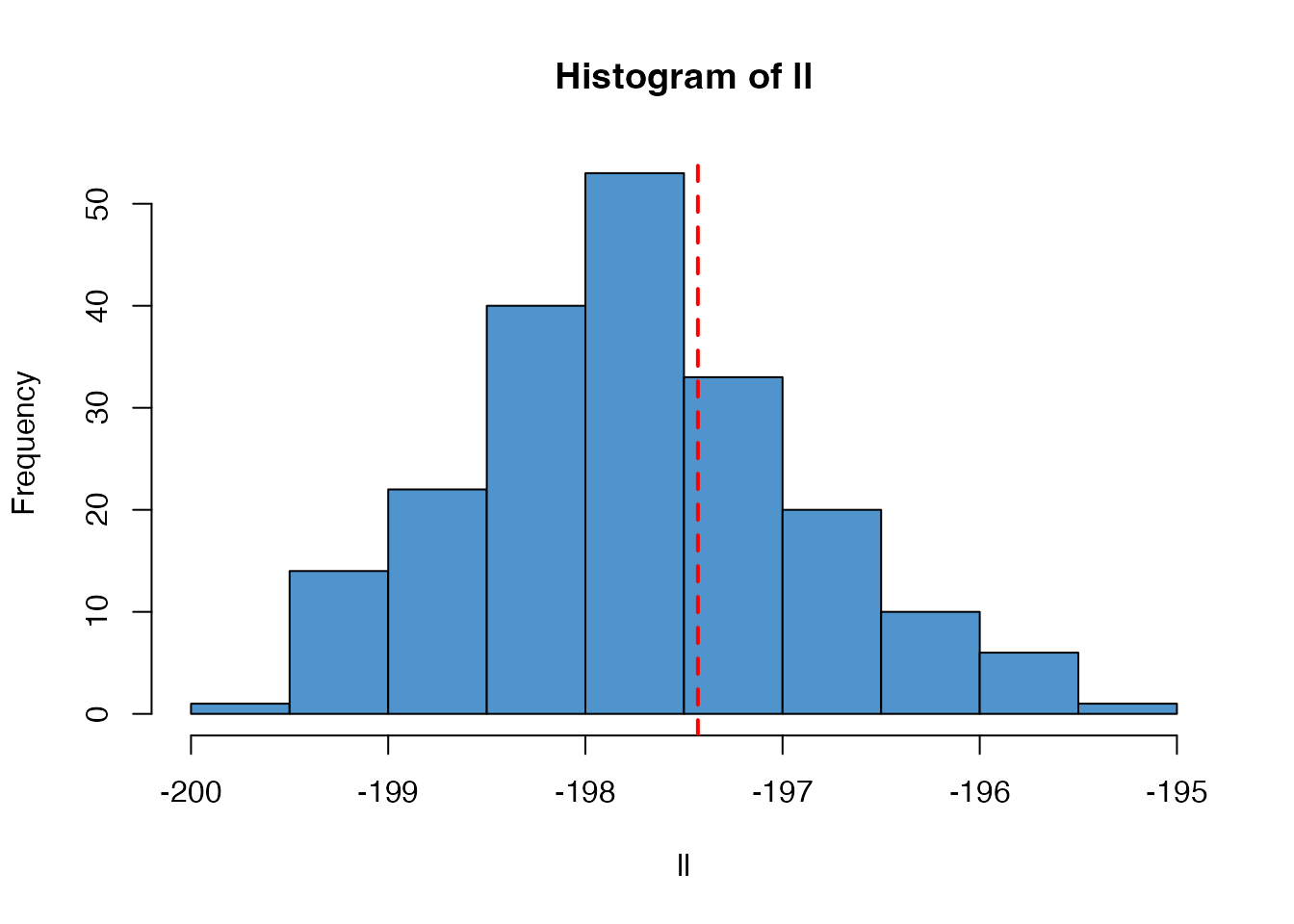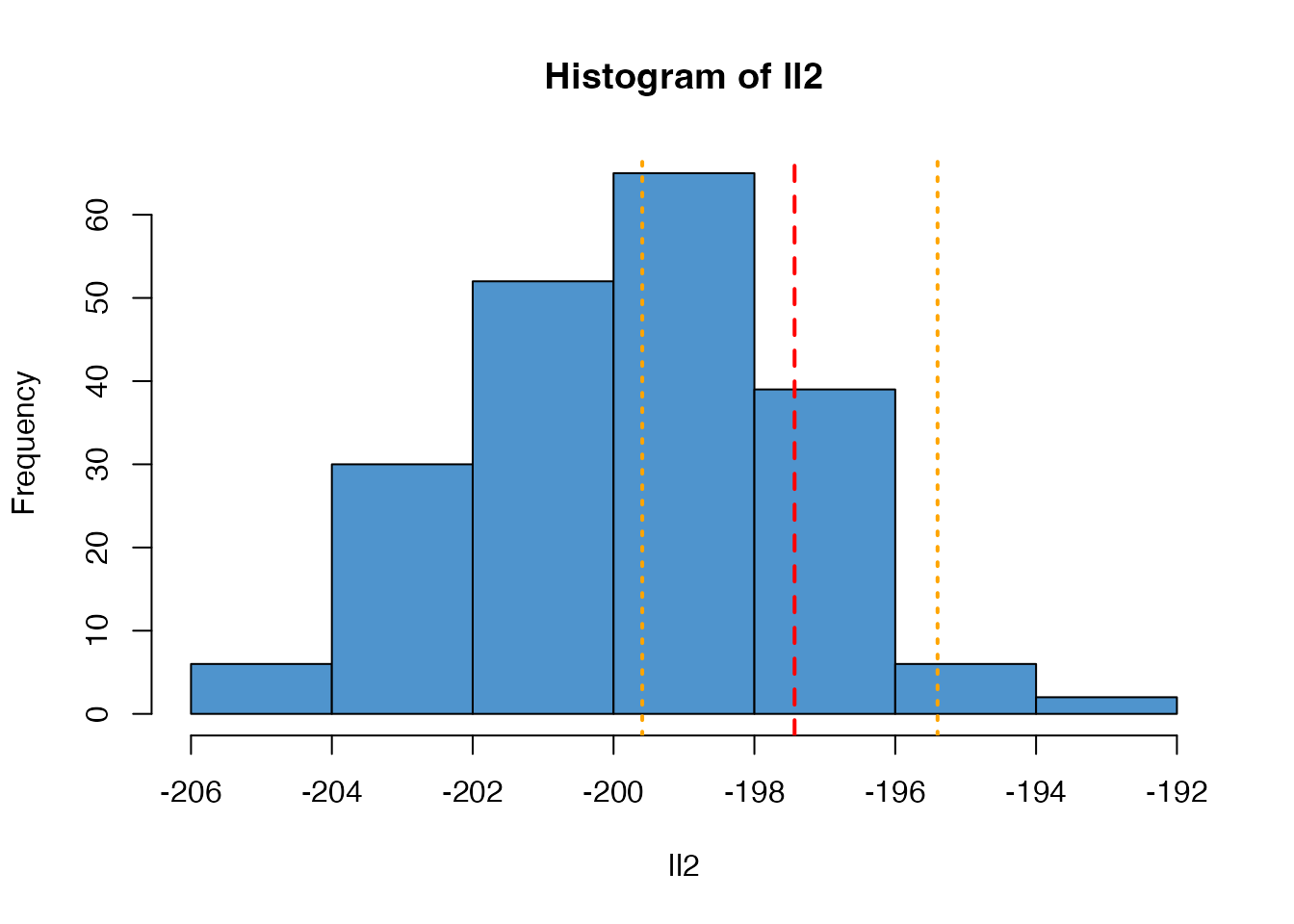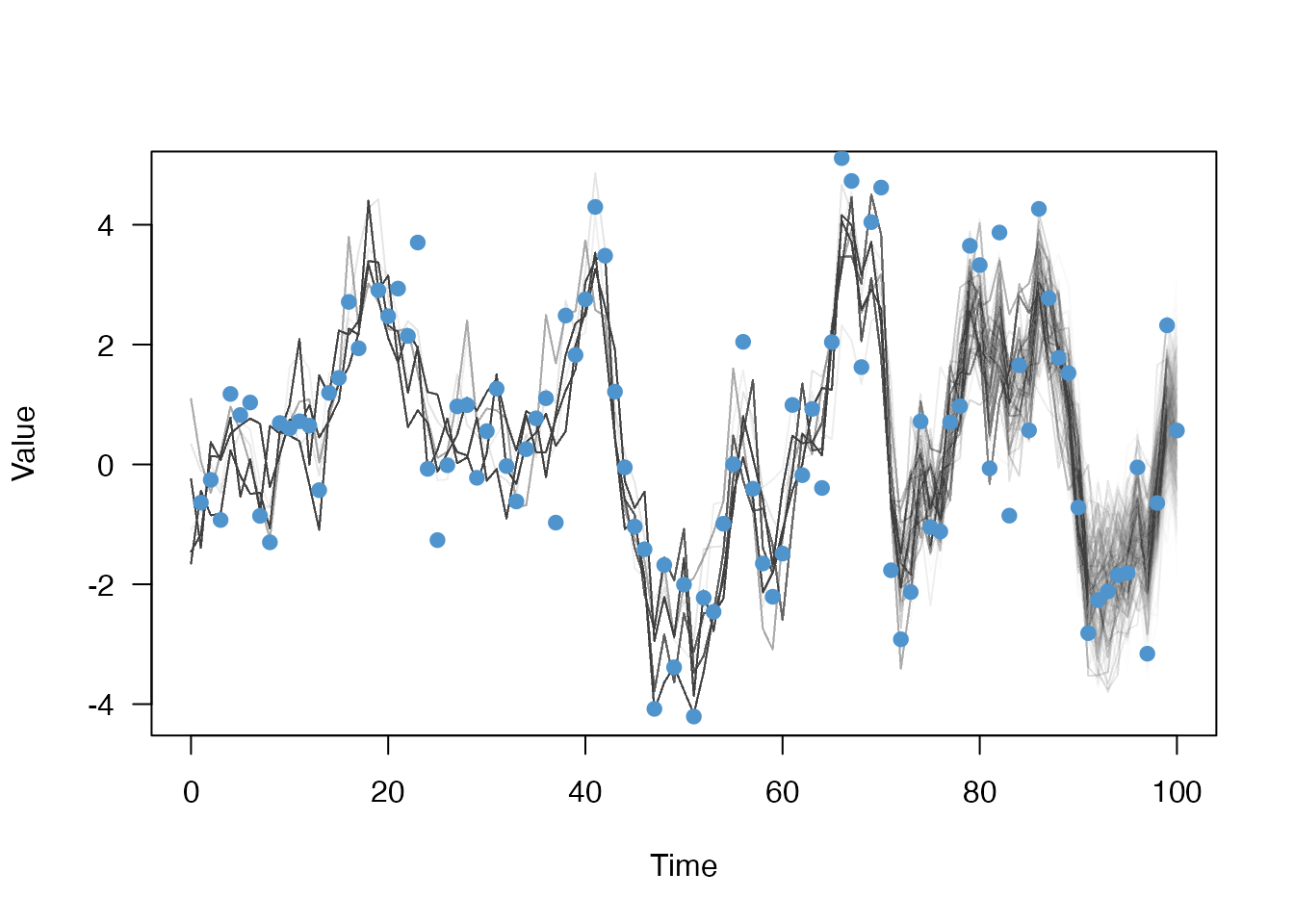We can test that the SMC algorithm implemented in mcstate::particle_filter() gives an unbiased estimate of the likelihood by comparing a simple model where the likelihood can be calculated exactly. The volatility model included in the dust package fulfils this criteria:

class volatility {
public:
using real_type = double;
using internal_type = dust::no_internal;
using rng_state_type = dust::random::generator<real_type>;

struct data_type {
real_type observed;
};

struct shared_type {
real_type alpha;
real_type sigma;
real_type gamma;
real_type tau;
real_type x0;
};

volatility(const dust::pars_type<volatility>& pars) : shared(pars.shared) {
}

size_t size() const {
return 1;
}

std::vector<real_type> initial(size_t time, rng_state_type& rng_state) {
std::vector<real_type> state(1);
state = shared->x0;
return state;
}

void update(size_t time, const real_type * state,
rng_state_type& rng_state, real_type * state_next) {
const real_type x = state;
state_next = shared->alpha * x +
shared->sigma * dust::random::normal<real_type>(rng_state, 0, 1);
}

real_type compare_data(const real_type * state, const data_type& data,
rng_state_type& rng_state) {
return dust::density::normal(data.observed, shared->gamma * state,
shared->tau, true);
}

private:
dust::shared_ptr<volatility> shared;
};

// Helper function for accepting values with defaults
inline double with_default(double default_value, cpp11::sexp value) {
return value == R_NilValue ? default_value : cpp11::as_cpp<double>(value);
}

namespace dust {

template <>
dust::pars_type<volatility> dust_pars<volatility>(cpp11::list pars) {
using real_type = volatility::real_type;
real_type x0 = 0;
real_type alpha = with_default(0.91, pars["alpha"]);
real_type sigma = with_default(1, pars["sigma"]);
real_type gamma = with_default(1, pars["gamma"]);
real_type tau = with_default(1, pars["tau"]);

volatility::shared_type shared{alpha, sigma, gamma, tau, x0};
return dust::pars_type<volatility>(shared);
}

template <>
volatility::data_type dust_data<volatility>(cpp11::list data) {
return volatility::data_type{cpp11::as_cpp<double>(data["observed"])};
}

}

This is a dust model; refer to the documentation there for details. This file can be compiled with dust::dust, but here we use the version bundled with dust

volatility <- dust::dust_example("volatility")

We can simulate some data from the model:

head(data)
#>   t      value
#> 1 1 -0.5303308
#> 2 2  0.2668607
#> 3 3  3.1610953
#> 4 4  0.5045106
#> 5 5  1.1061410
#> 6 6 -0.7972183
plot(value ~ t, data, type = "o", pch = 19, las = 1)In order to estimate the parameters of the process that might have generated this dataset, we need, in addition to our model, an observation/comparison function. In this case, given that we observe some state:

volatility_compare <- function(state, observed, pars) {
dnorm(observed$value, pars$gamma * drop(state), pars$tau, log = TRUE) } We also model the initialisation process: volatility_initial <- function(info, n_particles, pars) { matrix(rnorm(n_particles, 0, pars$sd), 1)
}

pars <- list(
# Generation process
alpha = 0.91,
sigma = 1,
# Observation process
gamma = 1,
tau = 1,
# Initial condition
sd = 1)

and preprocess the data into the correct format:

volatility_data <- mcstate::particle_filter_data(data, "t", 1)
#> Warning in mcstate::particle_filter_data(data, "t", 1): 'initial_time' should
#> be provided. I'm assuming '0' which is one time unit before the first time in
#> your data (1), but this might not be appropriate. This will become an error in
#> a future version of mcstate
#>   t_start t_end time_start time_end      value
#> 1       0     1          0        1 -0.5303308
#> 2       1     2          1        2  0.2668607
#> 3       2     3          2        3  3.1610953
#> 4       3     4          3        4  0.5045106
#> 5       4     5          4        5  1.1061410
#> 6       5     6          5        6 -0.7972183

The particle filter can run with more or fewer particles - this will trade-off runtime with the variance in the estimate, though in a fairly non-linear manner:

n_particles <- 1000

With all these pieces we can create our particle filter object with 1000 particles

filter <- mcstate::particle_filter$new(volatility_data, volatility, n_particles, compare = volatility_compare, initial = volatility_initial) filter #> <particle_filter> #> Public: #> has_multiple_data: FALSE #> has_multiple_parameters: FALSE #> history: function (index_particle = NULL) #> initialize: function (data, model, n_particles, compare, index = NULL, initial = NULL, #> inputs: function () #> model: dust_generator, R6ClassGenerator #> n_data: 1 #> n_parameters: 1 #> n_particles: 1000 #> ode_statistics: function () #> restart_state: function (index_particle = NULL) #> run: function (pars = list(), save_history = FALSE, save_restart = NULL, #> run_begin: function (pars = list(), save_history = FALSE, save_restart = NULL, #> set_n_threads: function (n_threads) #> state: function (index_state = NULL) #> Private: #> compare: function (state, observed, pars) #> constant_log_likelihood: NULL #> data: particle_filter_data_single, particle_filter_data_discrete, particle_filter_data, data.frame #> data_split: list #> gpu_config: NULL #> index: NULL #> initial: function (info, n_particles, pars) #> last_history: NULL #> last_model: NULL #> last_restart_state: NULL #> last_stages: NULL #> last_state: NULL #> n_threads: 1 #> ode_control: NULL #> seed: NULL #> stochastic_schedule: NULL #> times: 0 1 2 3 4 5 6 7 8 9 10 11 12 13 14 15 16 17 18 19 20 21 ... Running the particle filter simulates the process on all $$10^3$$ particles and compares at each timestep the simulated data with your observed data using the provided comparison function. It returns the log-likelihood: filter$run(pars)
#>  -194.4017

This is stochastic and each time you run it, the estimate will differ:

filter$run(pars) #>  -195.6955 In this case the model is simple enough that we can use a Kalman Filter to calculate the likelihood exactly: kalman_filter <- function(alpha, sigma, gamma, tau, data) { y <- data$value

mu <- 0
s <- 1
log_likelihood <- 0

for (t in seq_along(y)) {
mu <- alpha * mu
s <- alpha^2 * s + sigma^2
m <- gamma * mu

S <- gamma^2 * s + tau^2
K <- gamma * s / S

mu <- mu + K * (y[t] - m)
s <- s - gamma * K * s

log_likelihood <- log_likelihood + dnorm(y[t], m, sqrt(S), log = TRUE)
}

log_likelihood
}
ll_k <- kalman_filter(pars$alpha, pars$sigma, pars$gamma, pars$tau,
volatility_data)
ll_k
#>  -195.0466

Unlike the particle filter the Kalman filter is deterministic:

kalman_filter(pars$alpha, pars$sigma, pars$gamma, pars$tau, volatility_data)
#>  -195.0466

However, the particle filter, run multiple times, will create a distribution centred on this likelihood:

n_replicates <- 200
ll <- replicate(n_replicates, filter$run(pars)) hist(ll, col = "steelblue3") abline(v = ll_k, col = "red", lty = 2, lwd = 2)As the number of particles used changes, the variance of this estimate will change filter2 <- mcstate::particle_filter$new(volatility_data, volatility,
n_particles / 10,
compare = volatility_compare,
initial = volatility_initial)
ll2 <- replicate(n_replicates, filter2$run(pars)) hist(ll2, col = "steelblue3") abline(v = ll_k, col = "red", lty = 2, lwd = 2) abline(v = range(ll), col = "orange", lty = 3, lwd = 2)If you run a particle filter with save_history = TRUE, it will record the (filtered) trajectories:s filter$run(pars, save_history = TRUE)
#>  -194.6735
dim(filter$history()) #>  1 1000 101 This is a N state (here 1) x N particles (1000) x N time steps (100) 3d array, but we will drop the first rank of this for plotting matplot(0:100, t(drop(filter$history())), xlab = "Time", ylab = "Value",
las = 1, type = "l", lty = 1, col = "#00000002")
points(value ~ t, data, col = "steelblue3", pch = 19)